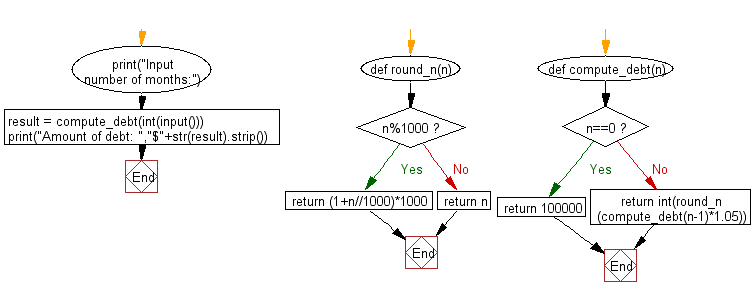﻿ Python: Compute the amount of the debt in n months - w3resource# Python: Compute the amount of the debt in n months

## Python Basic - 1: Exercise-36 with Solution

Write a Python program to compute the amount of the debt in n months. The borrowing amount is \$100,000 and the loan adds 5% interest of the debt and rounds it to the nearest 1,000 above month by month.

Input:
An integer n (0 ≤ n ≤ 100)
Input number of months: 7
Amount of debt: \$144000

Sample Solution:

Python Code:

``````def round_n(n):
if n%1000:
return (1+n//1000)*1000
else:
return n

def compute_debt(n):
if n==0: return 100000
return int(round_n(compute_debt(n-1)*1.05))

print("Input number of months:")
result = compute_debt(int(input()))
print("Amount of debt: ","\$"+str(result).strip())
``````

Sample Output:

```Input number of months:
7
Amount of debt:  \$144000
```

Flowchart:Python Code Editor:

Have another way to solve this solution? Contribute your code (and comments) through Disqus.

What is the difficulty level of this exercise?

Test your Programming skills with w3resource's quiz.

﻿

## Python: Tips of the Day

What is the difference between Python's list methods append and extend?

append: Appends object at the end.

```x = [1, 2, 3]
x.append([4, 5])
print (x)
```

Output:

```[1, 2, 3, [4, 5]]
```

extend: Extends list by appending elements from the iterable.

```x = [1, 2, 3]
x.extend([4, 5])
print (x)
```

Output:

```[1, 2, 3, 4, 5]
```

Ref: https://bit.ly/2AZ6ZFq